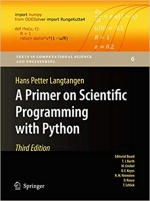# A Primer on Scientific Programming with Python (Texts in Computational Science and Engineering) 3rd ed. 2012 Edition by Hans Petter LangtangenКатегория: Other

Поделиться:
The book serves as a first introduction to computer programming of scientific applications, using the high-level Python language. The exposition is example- and problem-oriented, where the applications are taken from mathematics, numerical calculus, statistics, physics, biology, and finance. The book teaches "Matlab-style" and procedural programming as well as object-oriented programming. High school mathematics is a required background, and it is advantageous to study classical and numerical one-variable calculus in parallel with reading this book. Besides learning how to program computers, the reader will also learn how to solve mathematical problems, arising in various branches of science and engineering, with the aid of numerical methods and programming. By blending program

### Комментарии

Ничего не найдено.